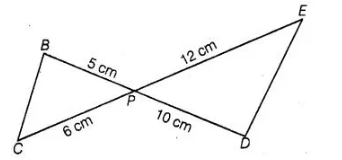# In figure, BD and CE intersect each

Question:

In figure, BD and CE intersect each other at the point P. Is ΔPBC ~ ΔPDE? Why?Solution:

True

$\angle B P C=\angle E P D$ [vertically opposite angles]

Now, $\frac{P B}{P D}=\frac{5}{10}=\frac{1}{2}$ $\ldots($ i)

and $\frac{P C}{P E}=\frac{6}{12}=\frac{1}{2}$ ...(ii)

From Eqs. (i) and (ii), $\frac{P B}{P D}=\frac{P C}{P E}$

Since, one angle of ΔPBC is equal to one angle of ΔPDE and the sides including these angles are proportional, so both triangles are similar.

Hence, ΔPBC ∼ ΔPDE, by SAS similarity criterion.